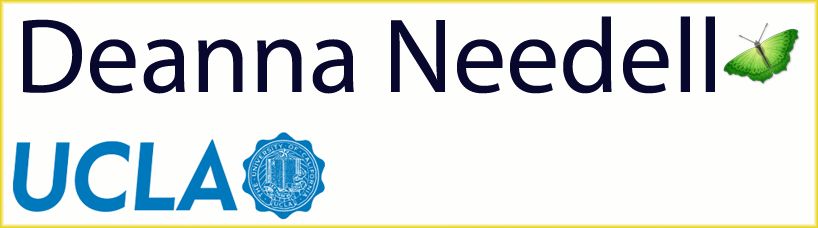# Teaching

Full courses taught listed here. For mentoring programs, see my Students page.

### UCLA

1. Math 170E (Probability), Instructor
2. Math 170A (Probability), Instructor
3. Math 270B (Mathematical Aspects of Scientific Computing: Computational Linear Algebra), Instructor
4. Math 270C (Mathematical Aspects of Scientific Computing: Computational Linear Algebra), Instructor
5. Math 285J (Seminar: Applied Mathematics Compressive sensing and large-scale techniques), Instructor
6. Math 285J (Seminar: Fairness in Machine Learning), Instructor

### Claremont McKenna College

1. Math 030 (Calculus I), Instructor
2. Math 052 (Introduction to Statistics), Instructor
3. CS 55 (Discrete Structures), Instructor
4. Math 151 (Probability), Instructor
5. Math 152 (Mathematical Statistics), Instructor
6. Math 168 (Wavelets and their Applications), Instructor
7. Math 169 (Representations of High Dimensional Data), Instructor

### Stanford

1. Stat 110 (Statistical Methods in Engineering and the Physical Sciences), Instructor
2. Stat 218 (Intro. to Stochastic Processes), Instructor
3. Stat 116 (Probability), Instructor

### UC Davis

1. Math 115A (Number Theory), Instructor
2. Math Circle: Games and Probability, Instructor
3. Math 108 (Abstract Math), Instructor
4. Math 21B (Calculus II), Lead Teaching Assistant
5. Math 16A (Calculus I), Lead Teaching Assistant
6. Math 280: Non-Asymptotic Random Matrix Theory, Teaching Assistant
7. Math 12 (Precalculus), Instructor

1. Math 124 (College Algebra), Instructor
2. Math 120 (Survey of Math), Instructor

### Sacramento City College

1. Math 100 (Beginning Algebra), Instructor
2. Math 120 (Intermediate Algebra), Instructor
3. Stat 300 (Probability and Statistics), Instructor

### Solano Comm. College

1. Math 104 (Intermediate Algebra), Instructor